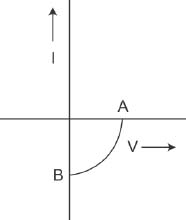#### The given graph represents V - I charachteristics for semincondutor device.Which of the following statement is correct? Option 1) It is V - I characteristics for solar cell where point A represents open circuit voltage and point B short circuit current. Option 2) It is for s solar cell and points A and B represent open circuit voltage and current, respectively. Option 3) It is for a photodiode and points A and B represent open circuit voltage and current, respectively. Option 4) It is for LED and points A and B represent open circuit voltage and short circuit current, respectively.V. I characteristics of a solar cell is shown. where A represent open circuit voltage (i.e I=0, V= Emf) and B shows short circuit voltage  (i.e I=I, V=0).

Option 1)

It is V - I characteristics for solar cell where point A represents open circuit voltage and point B short circuit current.

Correct option

Option 2)

It is for s solar cell and points A and B represent open circuit voltage and current, respectively.

Incorrect option

Option 3)

It is for a photodiode and points A and B represent open circuit voltage and current, respectively.

Incorrect option

Option 4)

It is for LED and points A and B represent open circuit voltage and short circuit current, respectively.

Incorrect option